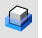HydrostaticsAnalyze

Mass Properties >

Hydrostatics

The Hydrostatics command reports hydrostatic values for surfaces and polysurfaces.

Hydrostatic values include wetted surface area, waterline length, maximum waterline beam, water plane area, and center of floatation.

Steps

• Select surfaces or polysurfaces.
Command-line options

WaterLineElevation

The water plane must always be horizontal in world coordinates. (This is a limitation of the command, not a statement of a physical principle.) Its location is defined by specifying the depth of the origin in world coordinates.

Symmetric

When only half of the model is given, the calculation results are doubled or adjusted as appropriate to represent the full model.

Longitude

The symmetry plane is either x=0 (when y is longitudinal) or y=0 (when x is longitudinal). The longitudinal direction (from bow to stern or front to back) must be either the direction of the x axis or the y axis.

Hydrostatics

Volume Displacement

Volume under the water.

Center of Buoyancy

Centroid of the volume displacement.

Wetted Surface Area

Surface area under water.

Waterline Length

Length at water line. The longitudinal bounding box extents of the water plane area.

Maximum Waterline Beam

Maximum beam at water line. The transverse bounding box extents of the water plane section.

Water Plane Area

Area of the cross section at the water plane.

Center of Floatation

Centroid of the water plane section These are the values for the whole model even if only a half model is given.

Copy All

Copies the information in the Hydrostatics box to the Clipboard.

Save As

Save the information to a file that you can use in spreadsheet programs.

Note

• To get displacement information there must be no naked edges below the waterline except in the case of Symmetric=Yes, in which case there can be naked edges on the symmetry plane.
• If the waterline falls on a singularity (place in the surface where points converge like at a pole of a sphere), the command will fail. Move the singularity point a fraction away from the water line.